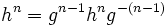# N-abelian implies every nth power and (n-1)th power commute

## Statement

Suppose$G$ is a group and$n$ is an integer such that$G$ is n-abelian: the$n^{th}$ power map on$G$ is an endomorphism of$G$. Then, for every$g,h \in G$ we have$h^ng^{n-1} = g^{n-1}h^n$.

## Related facts

### Other related facts

We say that a group is a n-abelian group if the$n^{th}$ power map is an endomorphism. Here are some related facts about$n$-abelian groups.

Value of$n$ (note that the condition for$n$ is the same as the condition for$1-n$) Characterization of$n$-abelian groups Proof Other related facts
0 all groups obvious
1 all groups obvious
2 abelian groups only 2-abelian iff abelian endomorphism sends more than three-fourths of elements to squares implies abelian
-1 abelian groups only -1-abelian iff abelian
3 3-abelian group means: 2-Engel group and derived subgroup has exponent dividing three Levi's characterization of 3-abelian groups cube map is surjective endomorphism implies abelian, cube map is endomorphism iff abelian (if order is not a multiple of 3), cube map is endomorphism implies class three
-2 same as for 3-abelian (based on n-abelian iff (1-n)-abelian)

## Facts used

1. Group acts as automorphisms by conjugation: For any$g \in G$, the map$c_g:x \mapsto gxg^{-1}$ is an automorphism of$G$.

## Proof

### From the power map being an endomorphism

Given: A group$G$ and a natural number$n$ such that$g \mapsto g^n$ is an endomorphism of$G$.

To prove: For every$g,h \in G$,$h^ng^{n-1} = g^{n-1}h^n$.

Proof: We denote by$c_g$ the map$x \mapsto gxg^{-1}$.

Step no. Assertion Facts used Given data used Previous steps used Explanation
1 For$g,h \in G$, we have$gh^ng^{-1} = (ghg^{-1})^n$ Fact (1) By fact (1),$c_g$ is an automorphism of$G$, so$c_g(h^n) = (c_g(h))^n$. Applying the definition of$c_g$ gives the result.
2 We have$(ghg^{-1})^n = g^nh^ng^{-n}$ for all$g,h \in G$.$n^{th}$ power map is endomorphism Since the$n^{th}$ power map is an endomorphism, we have$(abc)^n = a^nb^nc^n$. Setting$a = g, b = h, c = g^{-1}$ gives the desired result.
3 We get$h^ng^{n-1} = g^{n-1}h^n$ for all$g,h \in G$. In other words, every$n^{th}$ power commutes with every$(n - 1)^{th}$ power. Steps (1), (2) Combining steps (1) and (2) gives that$gh^ng^{-1} = g^nh^ng^{-n}$. Cancel a left-most$g$ and a right-most$g^{-1}$ on both sides, and get$h^n = g^{n-1}h^ng^{-(n-1)}$. Multiply both sides on the right by$g^{n-1}$ to get the desired result.

Thus, every$n^{th}$ power commutes with every$(n-1)^{th}$ power.